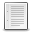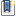File : Details

# linear.sci

a function for a partial inversion of a matrix
Details
Version
1.0
Author
robert coisson
Maintainer
robert coisson
Category
Supported Scilab Version
5.3
Creation Date
February 9, 2011Description
```            In a linear system Ax=b where A is a matrix and x,b are (column) vectors,
in case the unknowns are not all on one side, i.e. some x are known and others
unknown, and the same for b, this function program allows to calculate the
unknown ones.
The syntax is: [y,c]=linear(A,sigma_x,x,sigma_b,b)    where
sigma_x is a d-components column vector of the indices of the given x''s
sigma_b is a (n-d)-components column vector of the indices of the given b''s
x is a d-components column vector of the actual value of the given x''s
b is a (n-d)-components column vector of the actual value of the given b''s
Then [y,c] are the wanted solutions (the values of x,b which were unknown).
PS: the usual inversion of a linear system is recovered by
[y,c]=linear(A,[],[],[1:n]'',[b1;b2....;bn])            ```Files (1)Miscellaneous file
```by R. Coisson and G. Vernizzi
(see description below)
```News (0)Comments (1)Comment from Samuel Gougeon -- May 9, 2015, 11:08:05 PM
```Hello,

In order to prevent the global modification of mode() and ieee() user settings outside the
function, it would be nice coding this as follow:

mode_ini = mode()
mode(0)
ieee_ini = ieee()
ieee(1)
//... then, at the end, before quitting the function:
mode(mode_ini)
ieee(ieee_ini)

Thanks
Samuel
```Leave a comment
Login withEmail notifications